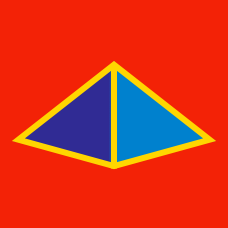Geometry

# Polyhedra: Level 2 Challenges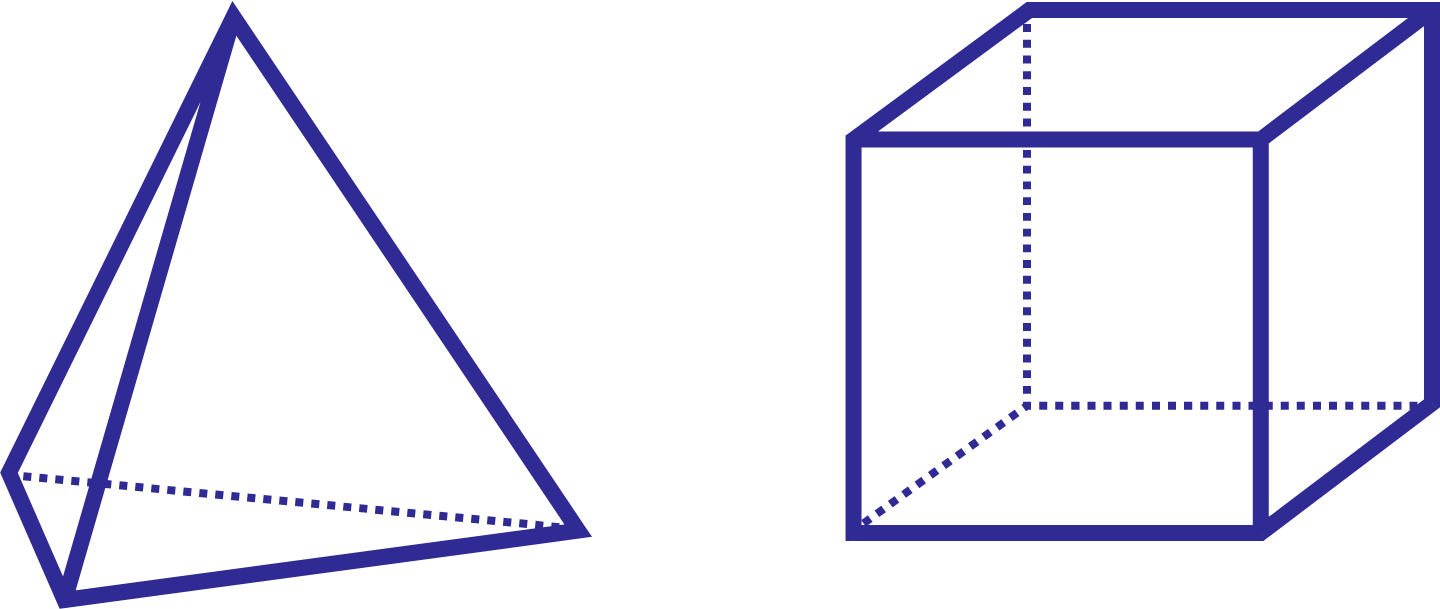Given a regular tetrahedron with volume $1 \text{cm}^3$ and a cube with volume $1 \text{cm}^3$, which object has smaller surface area?

Details and Assumptions:

• In a regular tetrahedron, all four faces are equilateral triangles, and

• In a cube, all six faces are squares.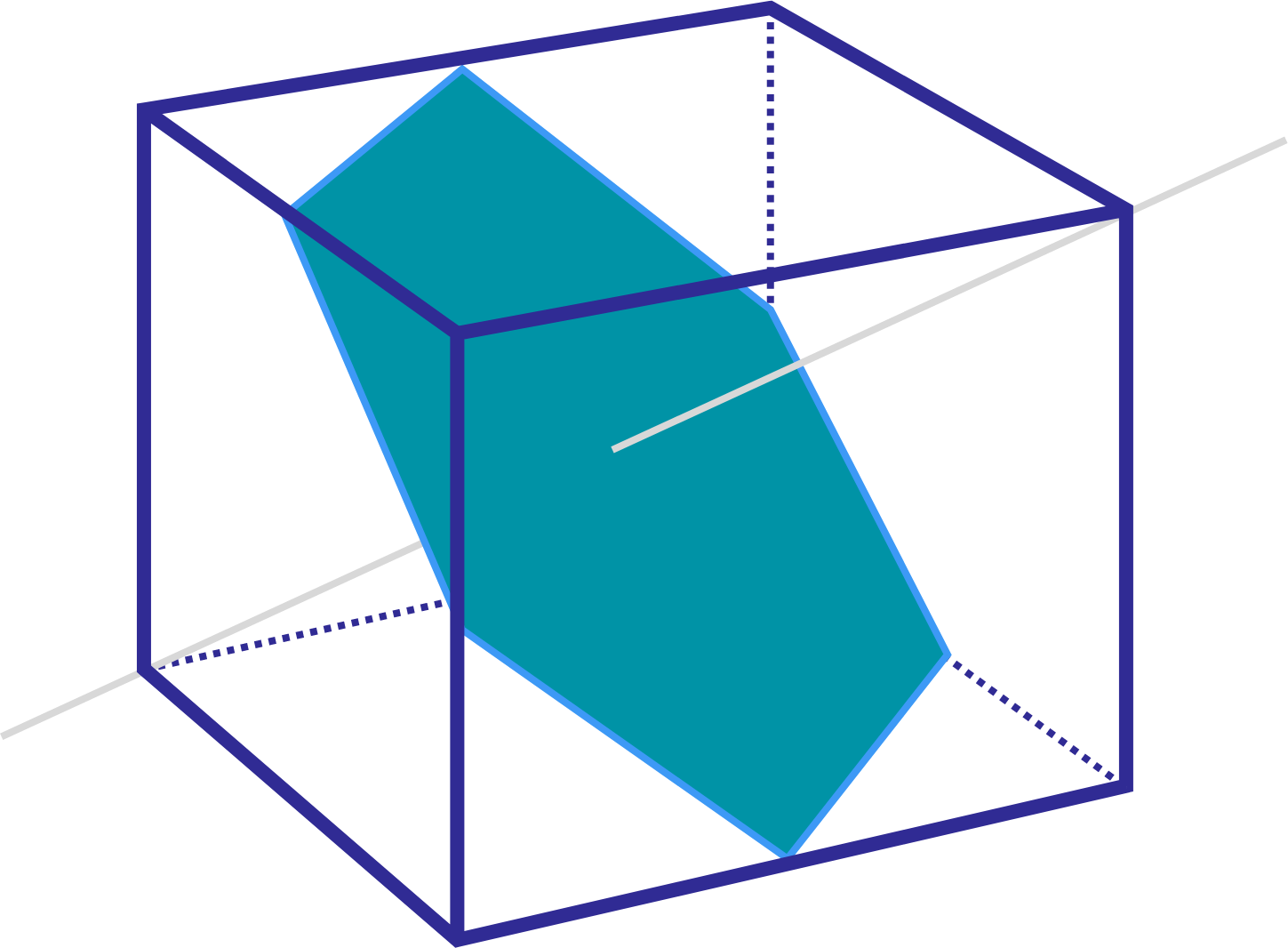The perfect slice through a cube will reveal a regular hexagonal cross section. If this is a $2\text{ in} \times 2\text{ in} \times 2\text{ in}$ cube, what is the surface area of the hexagonal cross section?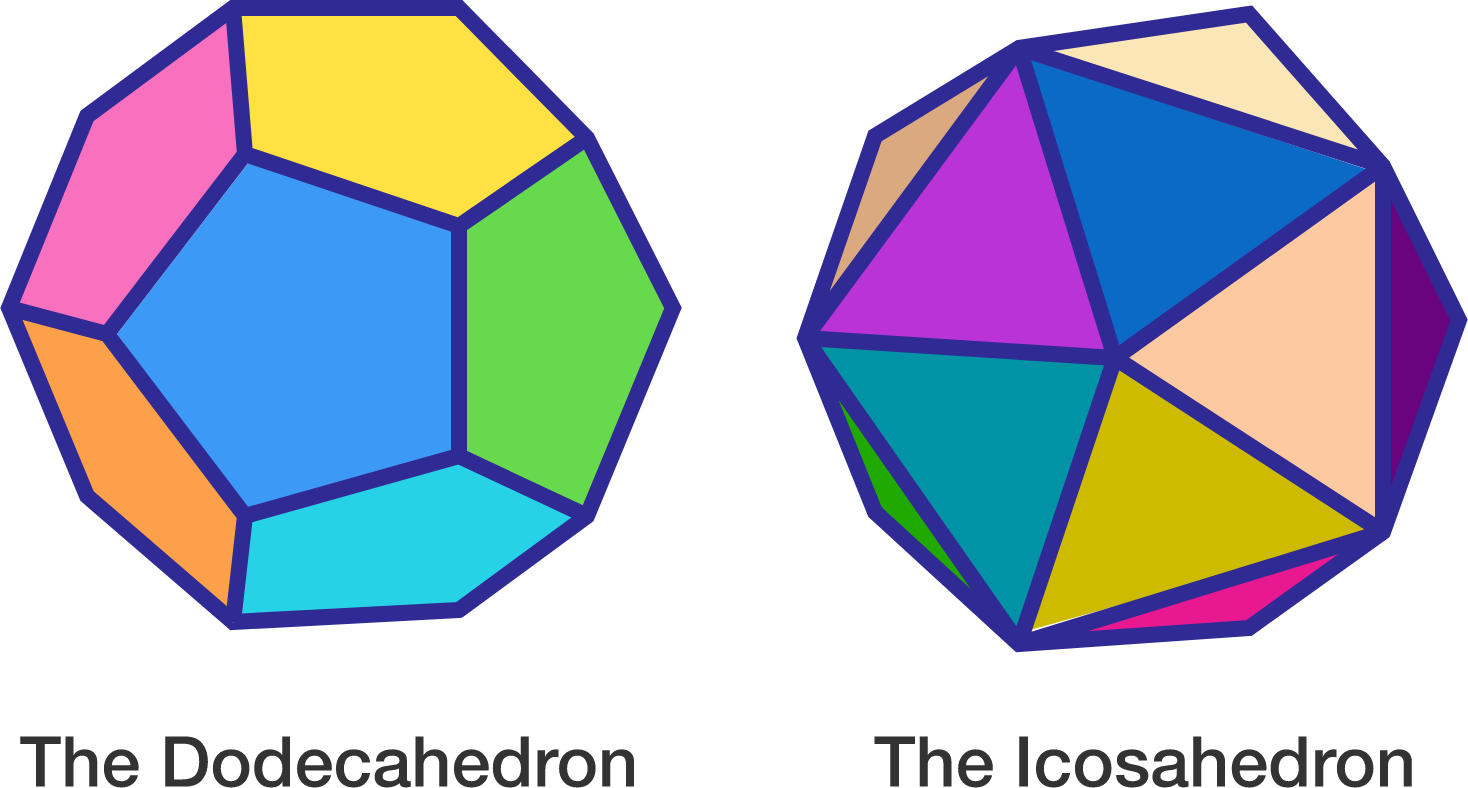The dodecahedron has 12 pentagonal faces. Therefore it has $\frac{12 \times 5}{2} = 30$ edges.

The icosahedron has 20 equilateral triangular faces. Therefore it has $\frac{20 \times 3}{2} = 30$ edges.

How many edges does a truncated icosahedron have?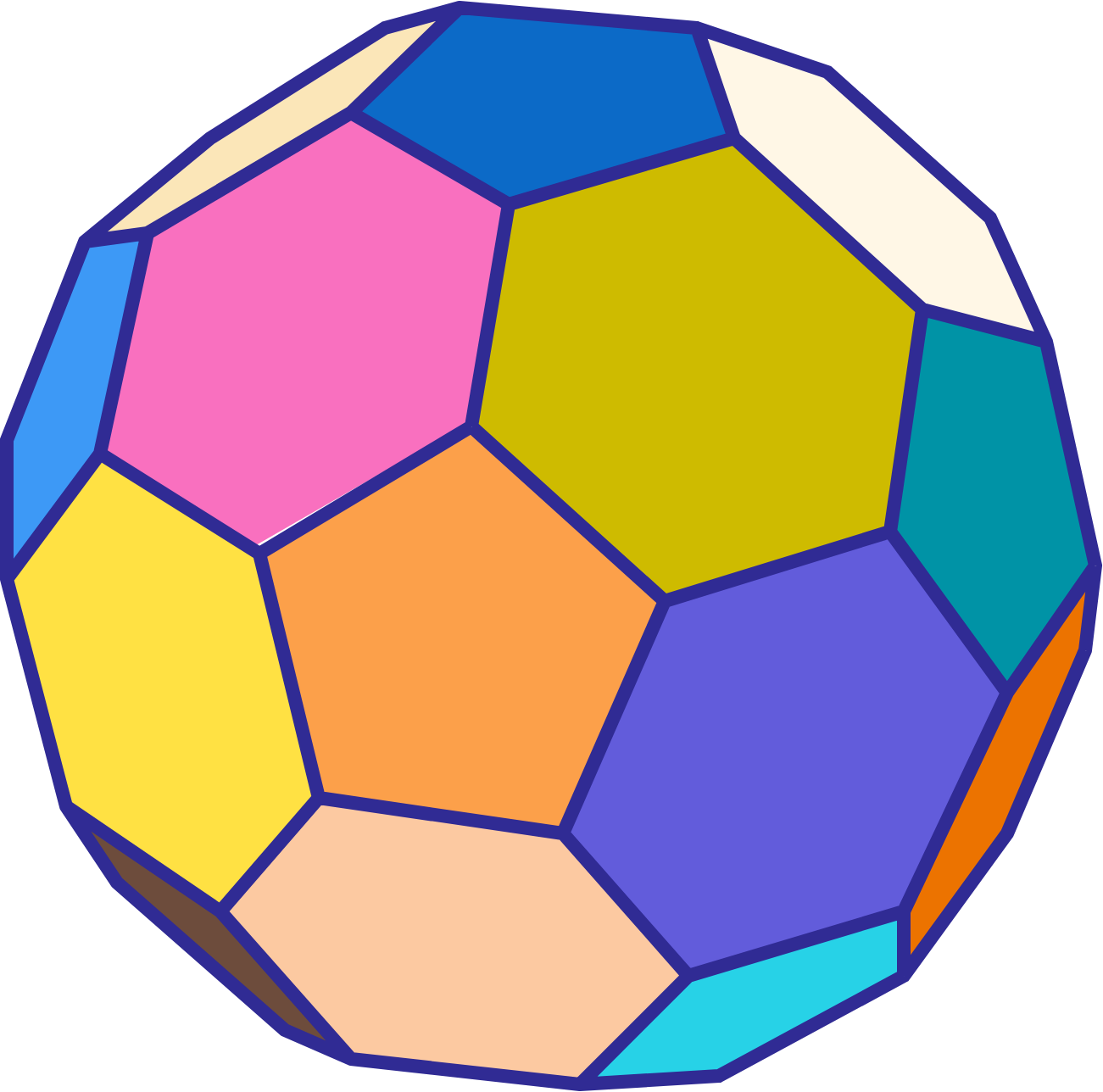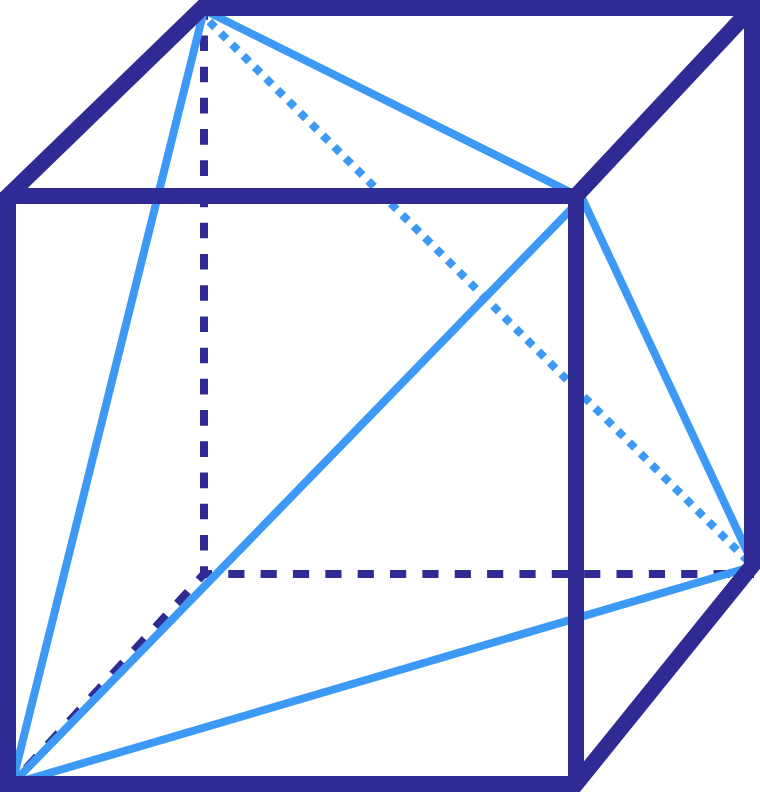After drawing in the diagonal perforations onto a unit cube, we are left with a geometric shape object. What is the volume of this new object?

A solid has 12 faces and 20 edges. Given that Euler's Formula applies, how many vertices does it have?

×

Problem Loading...

Note Loading...

Set Loading...